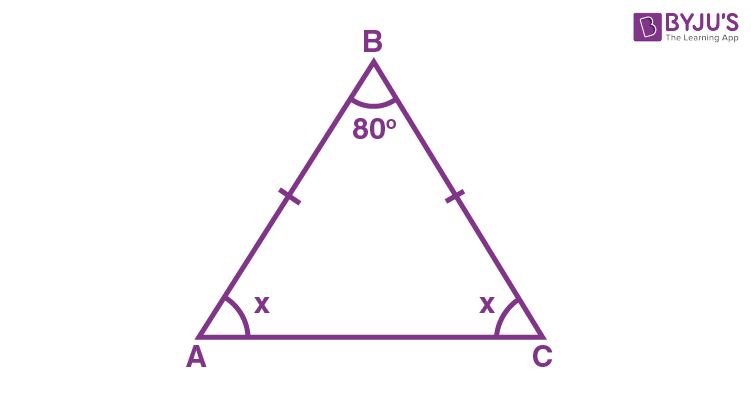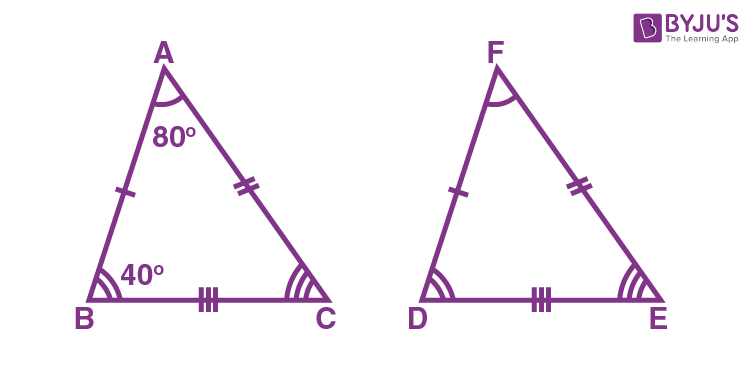# Class 9 Maths Chapter 7 Triangles MCQs

Class 9 Maths Chapter 7 Triangles MCQs are provided here with answers, online. Students can practice these objective questions to score good marks in the final exam. All the problems here are based on the CBSE syllabus and NCERT curriculum. A detailed explanation is given for each question to help students understand the concepts. Learn chapter-wise MCQs, only at BYJU’S. Also, check Important Questions for Class 9 Maths.

## MCQs on Class 9 Maths Chapter 7 Triangles

1) In triangle ABC, if AB=BC and ∠B = 70°, ∠A will be:

a. 70°

b. 110°

c. 55°

d. 130°

Explanation: Given,

AB = BC

Hence, ∠A=∠C

And ∠B = 70°

By angle sum property of triangle we know:

∠A+∠B+∠C = 180°

2∠A+∠B=180°

2∠A = 180-∠B = 180-70 = 110°

∠A = 55°

2) For two triangles, if two angles and the included side of one triangle are equal to two angles and the included side of another triangle. Then the congruency rule is:

a. SSS

b. ASA

c. SAS

d. None of the above

3) A triangle in which two sides are equal is called:

a. Scalene triangle

b. Equilateral triangle

c. Isosceles triangle

d. None of the above

4) The angles opposite to equal sides of a triangle are:

a. Equal

b. Unequal

c. supplementary angles

d. Complementary angles

5) If E and F are the midpoints of equal sides AB and AC of a triangle ABC. Then:

a. BF=AC

b. BF=AF

c. CE=AB

d. BF = CE

Explanation: AB and AC are equal sides.

AB = AC (Given)

∠A = ∠A (Common angle)

AE = AF (Halves of equal sides)

∆ ABF ≅ ∆ ACE (By SAS rule)

Hence, BF = CE (CPCT)

6) ABC is an isosceles triangle in which altitudes BE and CF are drawn to equal sides AC and AB respectively. Then:

a. BE>CF

b. BE<CF

c. BE=CF

d. None of the above

Explanation:

∠A = ∠A (common arm)

∠AEB = ∠AFC (Right angles)

AB = AC (Given)

∴ ΔAEB ≅ ΔAFC

Hence, BE = CF (by CPCT)

7) If ABC and DBC are two isosceles triangles on the same base BC. Then:

a. ∠ABD = ∠ACD

b. ∠ABD > ∠ACD

c. ∠ABD < ∠ACD

d. None of the above

AB = AC (Sides of isosceles triangle)

BD = CD (Sides of isosceles triangle)

So, ΔABD ≅ ΔACD.

∴ ∠ABD = ∠ACD (By CPCT)

8) If ABC is an equilateral triangle, then each angle equals to:

a. 90°

B.180°

c. 120°

d. 60°

Explanation: Equilateral triangle has all its sides equal and each angle measures 60°.

AB= BC = AC (All sides are equal)

Hence, ∠A = ∠B = ∠C (Opposite angles of equal sides)

Also, we know that,

∠A + ∠B + ∠C = 180°

⇒ 3∠A = 180°

⇒ ∠A = 60°

∴ ∠A = ∠B = ∠C = 60°

9) If AD is an altitude of an isosceles triangle ABC in which AB = AC. Then:

a. BD=CD

b. BD>CD

c. BD<CD

d. None of the above

Explanation: In ΔABD and ΔACD,

AB = AC (Given)

∴ ΔABD ≅ ΔACD (By RHS congruence condition)

BD = CD (By CPCT)

10) In a right triangle, the longest side is:

a. Perpendicular

b. Hypotenuse

c. Base

d. None of the above

Explanation: In triangle ABC, right-angled at B.

∠B = 90

By angle sum property, we know:

∠A + ∠B + ∠C = 180

Hence, ∠A + ∠C = 90

So, ∠B is the largest angle.

Therefore, the side (hypotenuse) opposite to the largest angle will be the longest one.

11) Which of the following is not a criterion for congruence of triangles?

(a) SAS

(b) ASA

(c) SSA

(d) SSS

Explanation:

SSA is not a criterion for the congruence of triangles. Whereas SAS, ASA and SSS are the criteria for the congruence of triangles.

12) In triangles ABC and PQR, AB = AC, ∠C = ∠P and ∠B = ∠Q. The two triangles are

(a) Isosceles and congruent

(b) Isosceles but not congruent

(c) Congruent but not isosceles

(d) Neither congruent nor isosceles

Explanation:

Consider two triangles, ABC and PQR. If the sides AB = AC and ∠C = ∠P and ∠B = ∠Q, then the two triangles are said to be isosceles, but they are not congruent.

13) In ∆ PQR, ∠R = ∠P and QR = 4 cm and PR = 5 cm. Then the length of PQ is

(a) 2 cm

(b) 2.5 cm

(c) 4 cm

(d) 5 cm

Explanation:

Given that, in a triangle PQR, ∠R = ∠P.

Since, ∠R = ∠P, the sides opposite to the equal angles are also equal.

Hence, the length of PQ is 4 cm.

14) If AB = QR, BC = PR and CA = PQ, then

(a) ∆ PQR ≅ ∆ BCA

(b) ∆ BAC ≅ ∆ RPQ

(c) ∆ CBA ≅ ∆ PRQ

(d) ∆ ABC ≅ ∆ PQR

Explanation:

Consider two triangles ABC and PQR.

Given that, AB = QR, BC = PR and CA = PQ.

By using Side-Side-Side (SSS rule),

We can say, ∆ CBA ≅ ∆ PRQ.

15) If ∆ ABC ≅ ∆ PQR, then which of the following is not true?

(a) AC = PR

(b) BC = PQ

(c) QR = BC

(d) AB = PQ

Explanation:

Given that, ∆ ABC ≅ ∆ PQR

Hence, AB = PQ

BC = QR

AC =PR

Thus, BC = PQ is not true, if ∆ ABC ≅ ∆ PQR.

16) In ∆ ABC, BC = AB and ∠B = 80°. Then ∠A is equal to

(a) 40°

(b) 50°

(c) 80°

(d) 100°

Explanation: In a triangle, ABC, BC = AB and ∠B = 80°.Thus, the given triangle is an isosceles triangle.

By using the angle sum property of a triangle, we get

x +  80°+x = 180°

2x+ 80°= 180°

2x = 180°- 80°

2x = 100°

x = 100°/2 = 50°

Therefore, ∠A = 50°.

17) Two sides of a triangle are of lengths 5 cm and 1.5 cm. The length of the third side of the triangle cannot be

(a) 3.4 cm

(b) 3.6 cm

(c) 3.8 cm

(d) 4.1 cm

Explanation: If two sides of a triangle are of lengths 5 cm and 1.5 cm, then the length of the third side of the triangle cannot be 3.4 cm. Because the difference between the two sides of a triangle should be less than the third side.

18) In ∆ ABC, AB = AC and ∠B = 50°. Then ∠C is equal to

(a) 40°

(b) 50°

(c) 80°

(d) 130°

Explanation:

Given that, in a triangle ABC, AB = AC and ∠B = 50°.

Since the given triangle is an isosceles triangle, the angles opposite to the equal sides are also equal. Hence, ∠C = 50°.

19) In ∆ PQR, if ∠R > ∠Q, then

(a) QR < PR

(b) PQ < PR

(c) PQ > PR

(d) QR > PR

Explanation: In a triangle PQR, if ∠R > ∠Q, then PQ > PR, because the side opposite to the greater angle is longer.

20) It is given that ∆ ABC ≅ ∆ FDE and AB = 5 cm, ∠B = 40° and ∠A = 80°. Then which of the following is true?

(a) DF = 5 cm, ∠F = 60°

(b) DF = 5 cm, ∠E = 60°

(c) DE = 5 cm, ∠E = 60°

(d) DE = 5 cm, ∠D = 40

Explanation:

Given that, ∆ ABC ≅ ∆ FDE and AB = 5 cm, ∠B = 40° and ∠A = 80°.By using the CPCT rule,

DF = 5 cm, since AB = 5 cm.

Again by using CPCT rule, ∠E = ∠C.

Therefore, ∠E = ∠C = 180° – (∠A+ ∠B) [ By using angle sum property of triangle]

∠E = 180° – (80°+ 40°)

∠E = 180° – 120°

∠E = 60°

Stay tuned with BYJU’S – The Learning App and download the app today to get more class-wise concepts.

1. Kartik Singh

This is a very good for teaching it’s vedios and teachers so amazing

2. K bhagya lakshmi

Apriciated and help full 😀😀😀

3. Saikumar

These questions are helpful for online tests

4. Brendan Churchill

Thank you bros Tomorrow is my maths mcq test Helped me lot Peace

6. Very helpful for multiple choice questions

7. so helpful tomorrow is my online test and this helped me a lot even the fear is gone thank you so much

8. Shubhankar Nanda

Very Very helpful mcqs for my exams

9. Piyush biswas

Thank you mams and sirs it is a very helpful

10. Piyush biswas

11. Thanks , It was very helpful for my exams

12. Archana

Myself Achu
Love from Kerala BYJU’S
Best doubt solving app ever
Thanks a million for your hardwork.

13. Gouri Nanda

14. ANUP NVC

THANK YOU IT IS VERY HELPFUL FOR MY TOMORROW MATHS EXAM

15. Komal Choudhary

Thank a lot for all your efforts

16. Angel Sharma

Doing Revision for finals 👍

17. Mehzabeen Begum

Thank u byju’s his question will help me in my online examination

18. Aishvarya sureshkumar

the mcqs are very helpful for my school exams. thank you so much byjus